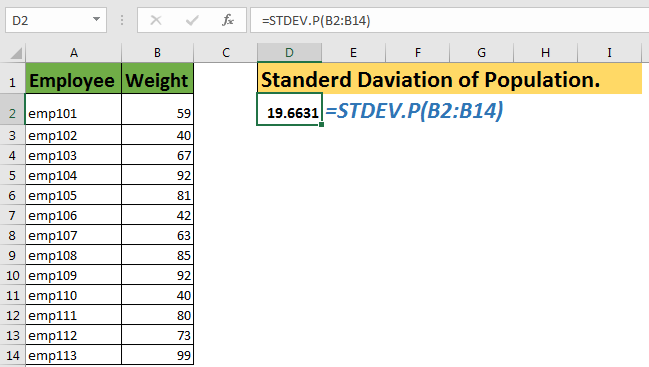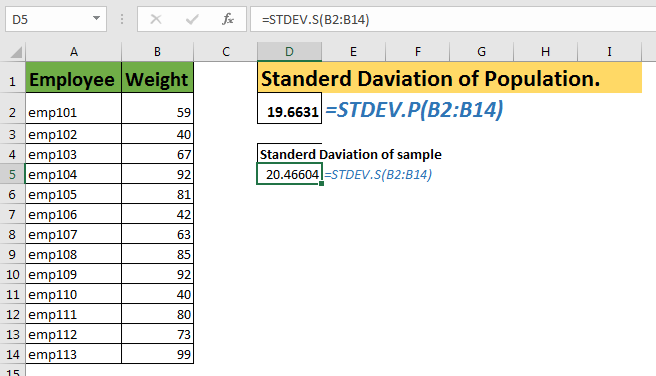# How To Use Excel STDEV.P FunctionEarlier, we learned about STDEV.S or STDEV function (both work the same). In this article, we will learn about STDEV.P function in Excel.
The STDEV.P is the latest version of the STDEVP function of excel. Both work same, but excel recommends STDEV.P function.
The STDEV.P function is used to calculate the standard deviation of the whole population. In fact, P in STDEVP stands for Population. If you have just a sample of data, then use STDEV.S function. The S in STDEVS stands for Sample.

Syntax of STDEV.P

=STDEV.P(number1,number2,...)

Number1,number2,...: these are the number of which you want to calculate the standard deviation.
The first number is compulsory.

The first argument is compulsory, and the rest are optional.
These numbers can be supplied as individual numbers, cell references, ranges or arrays to STDEV.P function.
All the below formulas are valid STDEV.P formulas.

=STDEV.P(1,2,3,{5,99})
=STDEV.P(A1,A2,B3,C2:C5)

Example Of STDEV.P Function
The below data tells the weight of each employee in the company. Now we need to calculate the standard deviation of the whole population. We are not taking any sample.
To calculate the standard deviation of weights of employees, we will write this STDEV.P formula.

=STDEV.P(B2:B14)

It will return the 19.66314
Now to see the difference between STDEV.P and STDEV.S, write this formula in a cell.

=STDEV.P(B2:B14)

It returns 20.46604442. It’s slightly larger then STDEV.P function’s output. Because the denominator in STDEV.S is N-1 here. Where in STDEV.P its N.How does it work?
The standard deviation of the population is evaluated when there is minimal risk to apply the result on aggregate data. Here we take the whole population for calculating the standard deviation. The sum of squared deviation is divided by N (number of arguments).
STDEV.P vs STDEV.S
So, what are the difference in STDEV.P and STDEV.S function? Let’s Explore.

The STDEV.P function is used when your data represents the entire population.
The STDEV.S function is used when your data is a sample of the entire population.

In STDEV.P function, the squared deviation is divided by the total number of arguments, mostly represented as N.
In STDEV.S or STDEV, the squared deviation is divided by the total number of sample -1. It is represented as N-1.

Since STDEV.P function considers entire data, and some factors may dominate the result standard deviation. And since it will be taken as the standard deviation for everyone in data, even for minorities, this is called Biased Analysis. This is why this standard deviation is recommended to use only when an analysis is non-destructive.
The STDEV.S or STDEV function is used on a small sample of the entire population, and we subtract one from the denominator (number of samples arguments). This is called non-biassed analysis of standard deviation. This is used when an analysis is destructive.

Important:

• The STDEV.P is just a newer version of STDEVP and STDEV functions of excel. There is no significant difference between them. However, Excel recommends the use of STDEV.P function
• The STDEV.P function only recognises numbers. Any other value is ignored.
• Use this function when you have captured the entire population. For sample data, use STDEV.S function.

So yeah, this STDEV.P function. I hope this was informative. If you have any doubts or suggestion regarding this article or any other function of excel, be my guest and use the comment section below. I will be more than happy to hear from you guys.

Related Data:
How to Use STDEV Function in Excel

How to Use STDEV.S Function in Excel

Regressions Analysis in Excel

How To Calculate MODE

How To Calculate Mean

How to Create a Standard Deviation Graph

Popular Articles:

50 Excel Shortcuts to Increase Your Productivity

How to use the VLOOKUP Function in Excel

How to use the COUNTIF in Excel 2016

How to use the SUMIF Function in Excel

Terms and Conditions of use

The applications/code on this site are distributed as is and without warranties or liability. In no event shall the owner of the copyrights, or the authors of the applications/code be liable for any loss of profit, any problems or any damage resulting from the use or evaluation of the applications/code.